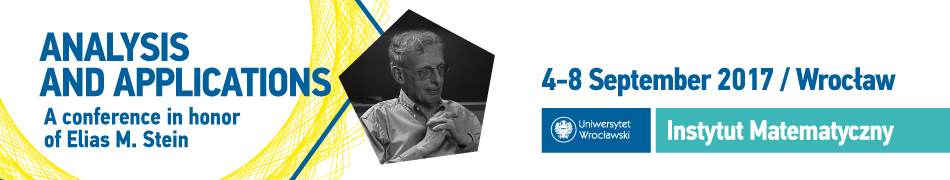Mariusz MirekRutgers University
Department of Mathematics
Hill Center-Busch Campus
Piscataway, NJ 08854-8019
USA

Contact:

Office: Hill Center 542
Phone: 848-445-6753
E-mail: mariusz.mirek[at]rutgers.edu

I am an Assistant Professor in the Department of Mathematics at Rutgers University and
I am an Associate Professor in the Mathematical Institute at the University of Wrocław.
I was a member of the School of Mathematics at the Institute for Advanced Study in Princeton.
I completed my PhD in Mathematics at the University of Wrocław in June 2011. I obtained my
Habilitation degree from the University of Bonn in June 2016 and from the University of Wrocław
in June 2017. My research interests are concentrated in the field of harmonic analysis and its
applications to ergodic theory and probability theory.

Education and employment:

• Assistant Professor in the Department of Mathematics at Rutgers University, (09.2018-Now).
• Lecturer in the Department of Mathematics at King's College London, (05.2017-09.2017).
• Member of the Institute for Advanced Study, Princeton, (09.2016-08.2017).
• Habilitation in Mathematics from the University of Wrocław, (20.06.2017).
• Habilitation in Mathematics from the University of Bonn, (08.06.2016).
• Associate Professor in the Mathematical Institute at the University of Wrocław, (10.2014-On leave).
• HCM Postdoctoral Research Fellowship at the University of Bonn, (10.2012-08.2016).
• Assistant Professor in the Mathematical Institute at the University of Wrocław, (10.2011-09.2014).
• PhD in Mathematics from the University of Wrocław, (07.06.2011).
• M.Sc. in Mathematics from the University of Wrocław, (05.09.2007).

Papers and preprints:

1. J. Bourgain, M. Mirek, E.M. Stein and B. Wróbel. On discrete Hardy--Littlewood maximal functions over the balls in $\mathbb Z^d$: dimension-free estimates.
Accepted for publication in the Geometric Aspects of Functional Analysis. Israel Seminar (GAFA). Lecture Notes in Mathematics.

2. J. Bourgain, M. Mirek, E.M. Stein and B. Wróbel. On the Hardy--Littlewood maximal functions in high dimensions: Continuous and discrete perspective.
Accepted for publication in the Geometric Aspects of Harmonic Analysis. A conference proceedings on the occasion of Fulvio Ricci's 70th birthday Cortona, Italy, 25-29.06.2018. Springer INdAM Series.

3. M. Mirek, E. M. Stein, P. Zorin-Kranich. Jump inequalities for translation-invariant polynomial averages and singular integrals on $\mathbb Z^d$.
Submitted.

4. M. Mirek, E. M. Stein, P. Zorin-Kranich. A bootstrapping approach to jump inequalities and their applications.
Accepted for publication in the Analysis & PDE.

5. M. Mirek, E. M. Stein, P. Zorin-Kranich. Jump inequalities via real interpolation.
Accepted for publication in the Mathematische Annalen.

6. J. Bourgain, M. Mirek, E.M. Stein and B. Wróbel. Dimension-free estimates for discrete Hardy-Littlewood averaging operators over the cubes in $\mathbb Z^d$.
American Journal of Mathematics 141, (2019), no. 4, 857-905.

7. M. Mirek, E. M. Stein and B. Trojan. $\ell^p(\mathbb Z^d)$-estimates for discrete operators of Radon type: Maximal functions and vector-valued estimates.
Journal of Functional Analysis 277, (2019), no. 8, 2471-2521.

8. J. Bourgain, M. Mirek, E.M. Stein and B. Wróbel. On dimension-free variational inequalities for averaging operators in $\mathbb R^d$.
Geometric And Functional Analysis (GAFA) 28, (2018), no. 1, 58-99.

9. M. Mirek. Square function estimates for discrete Radon transforms.
Analysis & PDE 11, (2018), no. 3, 583-608.

10. B. Krause, M. Mirek and B. Trojan. Two-parameter version of Bourgain's inequality I: Rational frequencies.
Advances in Mathematics 323, (2018), 720-744.

11. M. Mirek, E. M. Stein and B. Trojan. $\ell^p(\mathbb Z^d)$-estimates for discrete operators of Radon type: Variational estimates.
Inventiones Mathematicae 209, (2017), no. 3, 665-748.

12. M. Mirek, B. Trojan and P. Zorin-Kranich. Variational estimates for averages and truncated singular integrals along the prime numbers.
Transactions of the American Mathematical Society 369, (2017), no. 8, 5403-5423.

13. M. Mirek and C. Thiele. A local $T(b)$ theorem for perfect Calderón-Zygmund operators.
Proceedings of the London Mathematical Society 114, (2017), no. 3, 35-59.

14. B. Krause, M. Mirek and B. Trojan. On the Hardy-Littlewood majorant problem for arithmetic sets.
Journal of Functional Analysis 271, (2016), no. 1, 164-181.

15. M. Mirek and B. Trojan. Discrete maximal functions in higher dimensions and applications to ergodic theory.
American Journal of Mathematics 138, (2016), no. 6, 1495-1532.

16. M. Mirek and B. Trojan. Cotlar's ergodic theorem along the prime numbers.
Journal of Fourier Analysis and Applications 21, (2015), no. 4, 822-848.

17. M. Mirek. Weak type $(1,1)$ inequalities for discrete rough maximal functions.
Journal d'Analyse Mathematique 127, (2015), no. 1, 247-281.

18. M. Mirek. Roth's Theorem in the Piatetski-Shapiro primes.
Revista Matemática Iberoamericana 31, (2015), no. 2, 617-656.

19. M. Mirek. $\ell^p(\mathbb Z)$-boundedness of discrete maximal functions along thin subsets of primes and pointwise ergodic theorems.
Mathematische Zeitschrift 279, (2015), no. 1-2, 27-59.

20. M. Mirek. Discrete analogues in harmonic analysis: maximal functions and singular integral operators.
Mathematisches Forschungsinstitut Oberwolfach: Real Analysis, Harmonic Analysis and Applications, 20-26 July 2014.
DOI: 10.4171/OWR/2014/34, Rep. no. 34, (2014), 1893-1896.

21. D. Buraczewski, E. Damek, S. Mentemeier and M. Mirek. Heavy tailed solutions of multivariate smoothing transforms.
Stochastic Processes and their Applications 123, (2013), 1947-1986.

22. M. Mirek. On fixed points of a generalized multidimensional affine recursion.
Probability Theory and Related Fields (2013), 156, no. 3-4, 665-705.

23. E. Damek, S. Mentemeier, M. Mirek and J. Zienkiewicz. Convergence to stable laws for multidimensional stochastic recursions: the case of regular matrices.
Potential Analysis (2013), 38 no. 3, 683-697.

24. D. Buraczewski, E. Damek and M. Mirek. Asymptotics of stationary solutions of multivariate stochastic recursions with heavy tailed inputs and related limit theorems.
Stochastic Processes and their Applications 122, (2012), 42-67.

25. M. Mirek. Heavy tail phenomenon and convergence to stable laws for iterated Lipschitz maps.
Probability Theory and Related Fields 151, (2011), no. 3, 705-734.

26. M. Mirek. Convergence to stable laws and a local limit theorem for stochastic recursions.
Colloquium Mathematicum 118, (2010), 705-720.

Updated: August 20, 2019.